# Class 11 Maths NCERT Solutions for Chapter 11 Conic Sections Exercise 11.1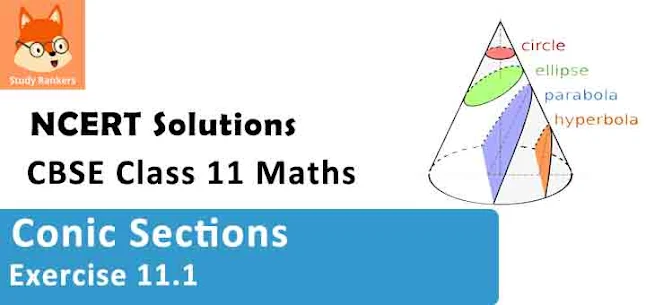### Conic Sections Exercise 11.1 Solutions

1.  Find the equation of the circle with centre (0, 2) and radius 2.

Solution

The equation of a circle with centre (h, k) and radius r is given as
(x­ – h)2 + (y ­– k)2 = r2
It is given that centre (h, k) = (0, 2) and radius (r) = 2.
Therefore, the equation of the circle is
(x – 0)2 + (y – 2)2 = 22
⇒ x2 + y2 + 4 ­– 4y = 4
⇒ x2 + y2 ­– 4y = 0

2. Find the equation of the circle with centre (–2, 3) and radius 4.

Solution

The equation of a circle with centre (h, k) and radius r is given as
(x­ – h)2 + (y ­– k)2 = r2
It is given that centre (h, k) = (–2, 3) and radius (r) = 4.
Therefore, the equation of the circle is
(x + 2)2 + (y – 3)2 = (4)2
⇒ x2 + 4x + 4 + y2 – 6y + 9 = 16
⇒ x2 + y2 + 4x – 6y – 3 = 0

3. Find the equation of the circle with (1/2, 1/4)and radius 1/12 .

Solution

The equation of a circle with centre (h, k) and radius r is given as
(x­ – h)2 + (y ­– k)2 = r2
It is given that centre (h, k) = (1/2, 1/4) and radius (r) = (1/12).
Therefore, the equation of the circle is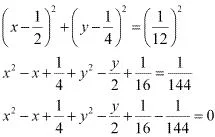⇒ 144x2 - 144x + 36 + 144y2 - 72y + 9 - 1 = 0
⇒ 144x2 - 144x + 144y2 - 72y + 44 = 0
⇒ 36x2 - 36x + 36y2 - 18y + 11 = 0
⇒ 36x2 + 36y2 - 36x - 18y + 11 = 0

4. Find the equation of the circle with centre (1, 1) and radius √2 .

Solution

The equation of a circle with centre (h, k) and radius r is given as
(x­ – h)2 + (y ­– k)2 = r2
It is given that centre (h, k) = (1, 1) and radius (r) = √2
Therefore, the equation of the circle is
(x - 1)2 + (y - 1)2 = (√2)2
⇒ x2 - 2x + 1 - y2 - 2y + 1 = 2
⇒ x2 + y2 - 2x - 2y = 0

5. Find the equation of the circle with centre (–a, –b) and radius √(a2 - b2)

Solution

The equation of a circle with centre (h, k) and radius r is given as
(x­ – h)2 + (y ­– k)2 = r2
It is given that centre (h, k) = (–a, –b) and radius (r) =.
Therefore, the equation of the circle is
(x + a)2 + (y + b)2 = [√(a2 - b2 )]2
⇒ x2 + 2ax + a2 + y2 + 2by + b2 = a2 - b2
⇒ x2 + y2 + 2ax + 2by + 2b2 = 0

6. Find the centre and radius of the circle (x + 5)2 + (y – 3)2 = 36

Solution

The equation of the given circle is (x + 5)2 + (y – 3)2 = 36.
(x + 5)2 + (y – 3)2 = 36
⇒ {x – (–5)}2 + (y – 3)2 = 62,
which is of the form (x – h)2 + (y – k)2 = r2, where h = –5, k = 3, and r = 6.
Thus, the centre of the given circle is (–5, 3), while its radius is 6.

7. Find the centre and radius of the circle x2 + y2 – 4x – 8y – 45 = 0

Solution

The equation of the given circle is x2 + y2 – 4x – 8y – 45 = 0.
x2 + y2 – 4x – 8y – 45 = 0
⇒ (x2 – 4x) + (y– 8y) = 45
⇒ {x2 – 2(x)(2) + 22} + {y2 – 2(y)(4)+ 42} – 4 –16 = 45
⇒ (x – 2)2 + (y –4)2 = 65
⇒ (x – 2)2 + (y –4)2 = (√65)2,
which is of the form (x – h)2 + (y – k)2 = r2, where h = 2, k = 4, and r = √65.
Thus, the centre of the given circle is (2, 4), while its radius is √65.

8. Find the centre and radius of the circle x2 + y2 – 8x + 10y – 12 = 0

Solution

The equation of the given circle is x2 + y2 – 8x + 10y – 12 = 0.
x2 + y2 – 8x + 10y – 12 = 0
⇒ (x2 – 8x) + (y+ 10y) = 12
⇒ {x2 – 2(x)(4) + 42} + {y+ 2(y)(5) + 52}– 16 – 25 = 12
⇒ (x – 4)2 + (y + 5)2 = 53
⇒ (x - 4)2 + [y - (-5)]2 = (√53)2 ,
which is of the form (x – h)2 + (y – k)2 = r2, where h = 4, k = –5, and r = √53 .
Thus, the centre of the given circle is (4, –5), while its radius is √53.

9. Find the centre and radius of the circle 2x2 + 2y2 – x = 0

Solution

The equation of the given circle is 2x2 + 2y2 – x = 0.
2x2 + 2y2 - x = 0
⇒ (2x2 - x) + 2y2 = 0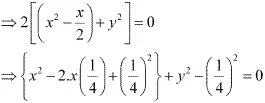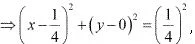which is of the form (x - h)2 + (y - k)2 = r2, where h = 1/4 , k = 0 and r = 1/4.
Thus, the centre of the given circle is (1/4, 0), while its radius is 1/4.

10. Find the equation of the circle passing through the points (4, 1) and (6, 5) and whose centre is on the line 4x + y = 16.

Solution

Let the equation of the required circle be (x – h)2 + (y – k)2 = r2.
Since the circle passes through points (4, 1) and (6, 5),
(4 – h)2 + (1 – k)2 = r2 …(1)
(6 – h)2 + (5 – k)2 = r2 …(2)
Since the centre (h, k) of the circle lies on line 4x + y = 16,
4h + k = 16 …(3)
From equations (1) and (2), we obtain
(4 – h)2 + (1 – k)= (6 – h)2 + (5 – k)2
⇒ 16 – 8h + h2 + 1 – 2k + k2 = 36 – 12h + h2 + 25 – 10k + k2
⇒ 16 – 8h + 1 – 2k = 36 – 12h + 25 – 10k
⇒ 4h + 8k = 44
⇒ h + 2k = 11 …(4)
On solving equations (3) and (4), we obtain h = 3 and k = 4.
On substituting the values of h and k in equation (1), we obtain
(4 – 3)2 + (1 – 4)2 = r2
⇒ (1)2 + (– 3)2 = r2
⇒ 1 + 9 = r2
⇒ r2 = 10
⇒ r = √10
Thus, the equation of the required circle is
(x – 3)2 + (y – 4)2 = (√10)2
x2 – 6x + 9 + y2 ­– 8y + 16 = 10
x2 + y2 – 6x – 8y + 15 = 0

11. Find the equation of the circle passing through the points (2, 3) and (–1, 1) and whose centre is on the line – 3y – 11 = 0.

Solution

Let the equation of the required circle be (x – h)2 + (y – k)2 = r2.
Since the circle passes through points (2, 3) and (–1, 1),
(2 – h)2 + (3 – k)2 = r2 …(1)
(–1 – h)2 + (1 – k)2 = r2 …(2)
Since the centre (h, k) of the circle lies on line x – 3y – 11 = 0,
h – 3k = 11 …(3)
From equations (1) and (2), we obtain
(2 – h)+ (3 – k)2 = (–1 – h)2 + (1 – k)2
⇒ 4 – 4h + h2 + 9 – 6k + k2 = 1 + 2h + h2 + 1 – 2k + k2
⇒ 4 – 4h + 9 – 6k = 1 + 2h + 1 – 2k
⇒ 6h + 4k = 11 …(4)
On solving equations (3) and (4), we obtain h = 7/2 and k = -5/2.
On substituting the values of h and k in equation (1), we obtain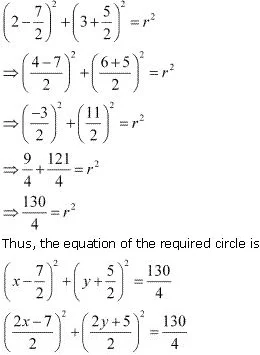4x2 - 28x + 49 + 4y2 + 20y + 25 = 130
⇒ 4x2 + 4y2 - 28x + 20y - 56 = 0
⇒ 4(x2 + y2 - 7x +5y - 14) = 0
⇒ x2 + y2 - 7x + 5y - 14 = 0

12. Find the equation of the circle with radius 5 whose centre lies on x-axis and passes through the point (2, 3).

Solution

Let the equation of the required circle be (x – h)2 + (y – k)2 = r2.
Since the radius of the circle is 5 and its centre lies on the x-axis, k = 0 and r = 5.
Now, the equation of the circle becomes (x – h)2 + y2 = 25.
It is given that the circle passes through point (2, 3).
∴ (2 - h)2 + 32 = 25
⇒ (2 - h)2 = 25 - 9
⇒ (2 - h)2 = 16
⇒ 2 - h = ± √16 = ± 4
If 2 - h = 4,  then h = -2.
If 2 - h = -4, then h = 6.
When h = -2, the equation of the circle becomes
(x + 2)2 + y2 = 25
⇒ x2 + 4x + 4 + y2 = 25
⇒ x2 + y2 + 4x – 21 = 0
When h = 6, the equation of the circle becomes
(x – 6)+ y2 = 25
⇒ x2 – 12x +36 + y2 = 25
⇒ x2 + y2 – 12x + 11 = 0

13. Find the equation of the circle passing through (0, 0) and making intercepts and b on the coordinate axes.

Solution

Let the equation of the required circle be (x – h)2 + (y – k)2 = r2.
Since the circle passes through (0, 0),
(0 – h)2 + (0 – k)2 = r2
⇒ h2 + k2 = r2
The equation of the circle now becomes (x – h)2 + (y – k)2 = h2 + k2.
It is given that the circle makes intercepts a and b on the coordinate axes. This means that the circle passes through points (a, 0) and (0, b). Therefore,
(a – h)2 + (0 – k)2 = h2 + k2 …(1)
(0 – h)2 + (b – k)2 = h2 + k2 …(2)
From equation (1), we obtain
a2 – 2ah + h2 + k2 = h2 + k2
⇒ a2 – 2ah = 0
⇒ a(a – 2h) = 0
⇒ a = 0 or (a – 2h) = 0
However, a ≠ 0; hence, (a – 2h) = 0 ⇒ h = a/2 .
From equation (2), we obtain
h2 + b2 – 2bk + k2 = h2 + k2
⇒ b2 – 2bk = 0
⇒ b(b – 2k) = 0
⇒ b = 0 or(b – 2k) = 0
However, b ≠ 0; hence, (b – 2k) = 0 ⇒ k = b/2.
Thus, the equation of the required circle is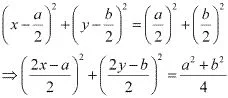⇒ 4x2 - 4ax + a2 + 4y2 - 4by + b2 = a2  + b2
⇒ 4x2 + 4y2 - 4ax - 4by = 0
⇒ x2 + y2 - ax - by = 0

14. Find the equation of a circle with centre (2, 2) and passes through the point (4, 5).

Solution

The centre of the circle is given as (h, k) = (2, 2).
Since the circle passes through point (4, 5), the radius (r) of the circle is the distance between the points (2, 2) and (4, 5).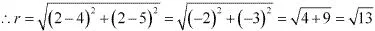Thus, the equation of the circle is
(x - h)2 + (y - k)2  = r2
⇒ (x - 2)2 + (y - 2)2 = (√13)2
⇒ x2 - 4x + 4 + y2 - 4y + 4 = 13
⇒ x2 + y2 - 4x - 4y - 5 = 0

15. Does the point (–2.5, 3.5) lie inside, outside or on the circle x2 + y2 = 25?

Solution

The equation of the given circle is x2 + y2 = 25.
x2 + y2 = 25
⇒ (x – 0)2 + (y – 0)2 = 52, which is of the form (x – h)2 + (y – k)2 = r2, where h = 0, k = 0, and r = 5.
∴ Centre = (0, 0) and radius = 5
Distance between point (–2.5, 3.5) and centre (0, 0)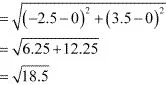= 4.3 (approx.) < 5
Since the distance between point (-2.5, 3.5) and centre (0, 0) of the circle is less than the radius of the circle, point (–2.5, 3.5) lies inside the circle.